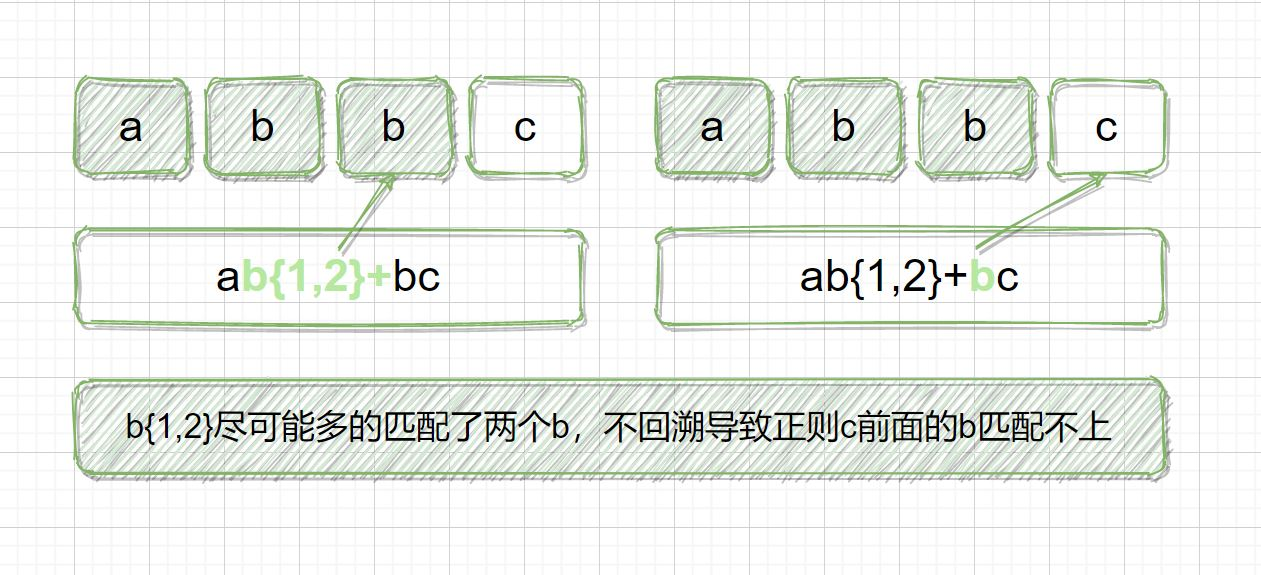# 正则表达式量词与贪婪的使用详解_正则表达式

##### 目录
0.写在前面1.量词2.贪婪模式前传2.1 使用 a+ 进行匹配2.2 使用 a* 进行匹配3.贪婪模式4.非贪婪模式5.独占模式5.1 贪婪匹配过程5.2 非贪婪匹配过程5.3 独占匹配过程6.写在最后

## 1.量词* {0,} ab*

a 或者 abb
+ {1,} ab+

ab 或者 abb

? {0,1} ab?

## 2.贪婪模式前传

### 2.1 使用 a+ 进行匹配```import re

print(re.findall(r'a+', 'aaabb'))

```

### 2.2 使用 a* 进行匹配```import re

print(re.findall(r'a*', 'aaabb'))

```

## 3.贪婪模式

a* 在匹配字符串 aaabb 时，会尽可能多的把前面的 a 都匹配上，直到第一个字母 b 不满足要求为止，匹配上3个 a，后面每次匹配的都是空字符串。

## 4.非贪婪模式```import re

// 贪婪匹配
print(re.findall(r'a*', 'aaabb'))

// 非贪婪匹配
print(re.findall(r'a*?', 'aaabb'))

```## 5.独占模式

### 5.1 贪婪匹配过程```import regex

print(regex.findall(r'ab{1,3}c', 'abbc'))

```

### 5.2 非贪婪匹配过程```import regex

print(regex.findall(r'ab{1,3}?c', 'abbc'))

```

### 5.3 独占匹配过程```import regex

print(regex.findall(r'ab{1,2}+bc', 'abbc'))

```

## 6.写在最后• 本文相关：
• python使用正则表达式去除(过滤)html标签提取文字功能
• 正则表达式之回溯
• java正则表达式获取大括号小括号内容并判断数字和小数亲测可用
• javascript正则表达式解析url的技巧
• 常用的正则表达式集锦
• idea 正则表达式搜索替换应用详解
• 正则表达式中最短匹配模式的用法浅析
• editplus中的正则表达式实战(6)
• php正则表达式的效率 回溯与固化分组
• 正则表达式 口诀 学习正则的朋友看看
• 详解java中的正则表达式
• PHP正则表达式的效率 回溯与固化分组
• 老生常谈JavaScript 正则表达式语法
• Python正则表达式操作指南
• python 正则表达式怎么匹配
• java 正则表达式的修正符写在哪
• 正则表达式?的用法
• 深入理解正则表达式语法知识
• javascript正则表达式总结
• 免责声明 - 关于我们 - 联系我们 - 广告联系 - 友情链接 - 帮助中心 - 频道导航